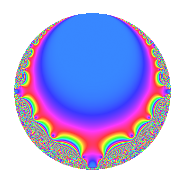# Properties

 Label 11.5.b.bLevel 11 Weight 5 Character orbit 11.b Analytic conductor 1.137 Analytic rank 0 Dimension 2 CM No Inner twists 2

# Related objects

## Newspace parameters

 Level: $$N$$ = $$11$$ Weight: $$k$$ = $$5$$ Character orbit: $$[\chi]$$ = 11.b (of order $$2$$ and degree $$1$$)

## Newform invariants

 Self dual: No Analytic conductor: $$1.13706959392$$ Analytic rank: $$0$$ Dimension: $$2$$ Coefficient field: $$\Q(\sqrt{-30})$$ Coefficient ring: $$\Z[a_1, a_2]$$ Coefficient ring index: $$1$$ Sato-Tate group: $\mathrm{SU}(2)[C_{2}]$

## $q$-expansion

Coefficients of the $$q$$-expansion are expressed in terms of $$\beta = \sqrt{-30}$$. We also show the integral $$q$$-expansion of the trace form.

 $$f(q)$$ $$=$$ $$q + \beta q^{2} -3 q^{3} -14 q^{4} + 31 q^{5} -3 \beta q^{6} -10 \beta q^{7} + 2 \beta q^{8} -72 q^{9} +O(q^{10})$$ $$q + \beta q^{2} -3 q^{3} -14 q^{4} + 31 q^{5} -3 \beta q^{6} -10 \beta q^{7} + 2 \beta q^{8} -72 q^{9} + 31 \beta q^{10} + ( 11 - 22 \beta ) q^{11} + 42 q^{12} + 34 \beta q^{13} + 300 q^{14} -93 q^{15} -284 q^{16} -42 \beta q^{17} -72 \beta q^{18} + 18 \beta q^{19} -434 q^{20} + 30 \beta q^{21} + ( 660 + 11 \beta ) q^{22} + 277 q^{23} -6 \beta q^{24} + 336 q^{25} -1020 q^{26} + 459 q^{27} + 140 \beta q^{28} + 232 \beta q^{29} -93 \beta q^{30} -1363 q^{31} -252 \beta q^{32} + ( -33 + 66 \beta ) q^{33} + 1260 q^{34} -310 \beta q^{35} + 1008 q^{36} + 167 q^{37} -540 q^{38} -102 \beta q^{39} + 62 \beta q^{40} + 194 \beta q^{41} -900 q^{42} -220 \beta q^{43} + ( -154 + 308 \beta ) q^{44} -2232 q^{45} + 277 \beta q^{46} + 1702 q^{47} + 852 q^{48} -599 q^{49} + 336 \beta q^{50} + 126 \beta q^{51} -476 \beta q^{52} + 4522 q^{53} + 459 \beta q^{54} + ( 341 - 682 \beta ) q^{55} + 600 q^{56} -54 \beta q^{57} -6960 q^{58} -2363 q^{59} + 1302 q^{60} -724 \beta q^{61} -1363 \beta q^{62} + 720 \beta q^{63} + 3016 q^{64} + 1054 \beta q^{65} + ( -1980 - 33 \beta ) q^{66} -2803 q^{67} + 588 \beta q^{68} -831 q^{69} + 9300 q^{70} + 3397 q^{71} -144 \beta q^{72} + 606 \beta q^{73} + 167 \beta q^{74} -1008 q^{75} -252 \beta q^{76} + ( -6600 - 110 \beta ) q^{77} + 3060 q^{78} + 1112 \beta q^{79} -8804 q^{80} + 4455 q^{81} -5820 q^{82} -152 \beta q^{83} -420 \beta q^{84} -1302 \beta q^{85} + 6600 q^{86} -696 \beta q^{87} + ( 1320 + 22 \beta ) q^{88} -4673 q^{89} -2232 \beta q^{90} + 10200 q^{91} -3878 q^{92} + 4089 q^{93} + 1702 \beta q^{94} + 558 \beta q^{95} + 756 \beta q^{96} + 4247 q^{97} -599 \beta q^{98} + ( -792 + 1584 \beta ) q^{99} +O(q^{100})$$ $$\operatorname{Tr}(f)(q)$$ $$=$$ $$2q - 6q^{3} - 28q^{4} + 62q^{5} - 144q^{9} + O(q^{10})$$ $$2q - 6q^{3} - 28q^{4} + 62q^{5} - 144q^{9} + 22q^{11} + 84q^{12} + 600q^{14} - 186q^{15} - 568q^{16} - 868q^{20} + 1320q^{22} + 554q^{23} + 672q^{25} - 2040q^{26} + 918q^{27} - 2726q^{31} - 66q^{33} + 2520q^{34} + 2016q^{36} + 334q^{37} - 1080q^{38} - 1800q^{42} - 308q^{44} - 4464q^{45} + 3404q^{47} + 1704q^{48} - 1198q^{49} + 9044q^{53} + 682q^{55} + 1200q^{56} - 13920q^{58} - 4726q^{59} + 2604q^{60} + 6032q^{64} - 3960q^{66} - 5606q^{67} - 1662q^{69} + 18600q^{70} + 6794q^{71} - 2016q^{75} - 13200q^{77} + 6120q^{78} - 17608q^{80} + 8910q^{81} - 11640q^{82} + 13200q^{86} + 2640q^{88} - 9346q^{89} + 20400q^{91} - 7756q^{92} + 8178q^{93} + 8494q^{97} - 1584q^{99} + O(q^{100})$$

## Character Values

We give the values of $$\chi$$ on generators for $$\left(\mathbb{Z}/11\mathbb{Z}\right)^\times$$.

 $$n$$ $$2$$ $$\chi(n)$$ $$-1$$

## Embeddings

For each embedding $$\iota_m$$ of the coefficient field, the values $$\iota_m(a_n)$$ are shown below.

For more information on an embedded modular form you can click on its label.

Label $$\iota_m(\nu)$$ $$a_{2}$$ $$a_{3}$$ $$a_{4}$$ $$a_{5}$$ $$a_{6}$$ $$a_{7}$$ $$a_{8}$$ $$a_{9}$$ $$a_{10}$$
10.1
 − 5.47723i 5.47723i
5.47723i −3.00000 −14.0000 31.0000 16.4317i 54.7723i 10.9545i −72.0000 169.794i
10.2 5.47723i −3.00000 −14.0000 31.0000 16.4317i 54.7723i 10.9545i −72.0000 169.794i
 $$n$$: e.g. 2-40 or 990-1000 Significant digits: Format: Complex embeddings Normalized embeddings Satake parameters Satake angles

## Inner twists

Char. orbit Parity Mult. Self Twist Proved
1.a Even 1 trivial yes
11.b Odd 1 yes

## Hecke kernels

This newform can be constructed as the kernel of the linear operator $$T_{2}^{2} + 30$$ acting on $$S_{5}^{\mathrm{new}}(11, [\chi])$$.